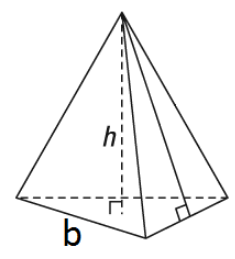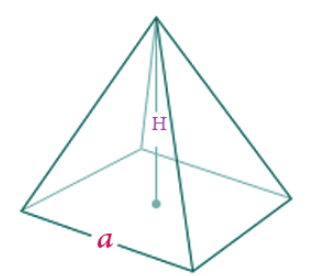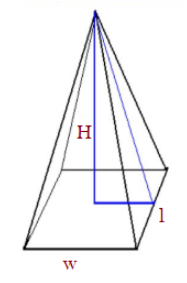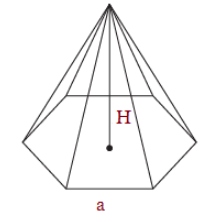# Volume of a Pyramid

The volume of a pyramid depends upon the type of pyramid’s base, whether it is a triangle, square or rectangle. A pyramid is a polyhedron figure which has only one base. The base of the pyramid is a poly sided figure. Hence, the formula to find not only volume but also the surface area of a pyramid will be based on the structure of its base and height of the pyramid.

Now let us find out the formula for the triangular pyramid, square pyramid and rectangular pyramid in this article.

## Derivation of Volume of a Pyramid

To find the volume of a pyramid, we need to know the total capacity of the given pyramid. The formula for the pyramid’s volume is given by one-third of the product of the area of the base to its height. Such as:

Volume = 1/3 x Area of the Base x Height

 V = ⅓ A × H

Where V = Volume, A = Area and H = height

Its volume is measured in the following units:

• in3
• ft3
• cm3
• m3 etc

Note: Always make sure to keep all the measurement units same while calculating the volume.

Now let us find out the formula for the volume of different types of pyramids.

## Volume of Pyramid Formula

Pyramids are of different types, such as:

• Triangular Pyramid
• Square Pyramid
• Rectangular Pyramid
• Hexagonal Pyramid

For all these types of pyramids, the formula for volume is different. Let us learn here all the volume formulas.

### Volume of Triangular Pyramid

A triangular pyramid has a base in triangle shape. As we know, the area of a triangle;

A = 1/2 b x h

where b is the base of the triangle and h is the altitude.Therefore, the volume of a triangular pyramid;

V = 1/3 x Area of triangular base x Height of pyramid

V = 1/3 x (1/2 bh) H

V = 1/6 bhH

### Volume of Square Pyramid

A square-based pyramid has a base in square shape. As we know, the area of a square is given by;

A = a2

Where a is the length of the side of the square.Hence, the volume of a square-sided pyramid is;

V = 1/3 x Area of square base x Height

V = 1/3 x a2 x H

V = 1/3 a2 H

### Volume of the Rectangular Pyramid

A rectangular pyramid has the base in a rectangular shape. Since, we know that the area of rectangle is equal to the product of its length and width, such as;

A = Length x Width

A = lwHence, the volume of a rectangular pyramid is given by;

V = 1/3 x A x H

V = 1/3 lwH

### Volume of Hexagonal Pyramid

The volume of a hexagonal pyramid, whose base is a regular hexagon shape is given by:

Volume = 1/3 x area of base x heightV= 1/3 x 3√3/2 a2 x H

V = √3/2 a2 H

Where a is the side length of the hexagon base and H is the height of the pyramid.

### Examples

Question 1: What is the volume of a pyramid whose base is square? The sides of the base are 10 cm each and the height of the pyramid is 18 cm.

Solution: To find the volume of a pyramid, We will use the formula – V = ⅓ A H

As the base of the pyramid is a square, the area of the base is a2 = 10 x 10 = 100 cm2

= ⅓ x 100 cm 2 x 18 cm

= 100 x 6

= 600 cm3

Question 2: What is the volume of a pyramid whose base is square? The sides of the base are 12 cm each and the height is 21 cm.

Solution: To find the volume of a pyramid, We will use the formula – V = ⅓ A H

As the base of the pyramid is a square, the area of the base is a2 = 12 x 12 = 144 cm2

= ⅓ x 144 cm 2 x 21 cm

= 144 x 7

= 1008 cm3

Question 3: If the base of the pyramid is rectangular having length is 7 cm and the width is 5 cm and the height of the pyramid is 10 cm, then find its volume.

Solution: Given, length of rectangular base of pyramid = 7 cm

width = 5 cm

and height of the pyramid = 10 cm

We know, the volume of rectangular pyramid,

V = 1/3 l w H

Substituting the values we get;

V = 1/3 x 7 x 5 x 10

V = 350/3 = 116.66 cubic.cm.

Question 4: For the triangular pyramid, the area of the base is 135 cubic cm. Find its volume if the height of pyramid is 7 cm.

Solution: Given the area of the base of pyramid = 135 cu.cm

Volume of Pyramid = 1/3 x A x H

V = 1/3 x 135 x 7

V = 45 x 7

V = 315 Cu. Cm.

Register at BYJU’S to learn the other mathematical topics in a fun and interesting way.

Test your knowledge on Volume Of A Pyramid